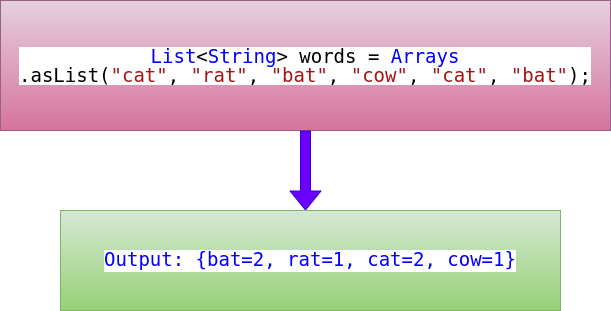## Posts

Showing posts with the label Java Programs for Beginners

### Java - How to Count the Number of Occurrences of Substring in a String

In this section, we will write a Java program to Count the Number of Occurrences of Substring in a String. Java program to Count the Number of Occurrences of Substring in a String In the below program, we have countOccurrencesOf(String str, String sub) a generic method, here we simply pass input string and substring as arguments and method return number of occurrences of the substring. /** * * Java program to count number of occurrence of substring in given string. * @author knowledgefactory.net * */ public class Main { public static void main ( String [] args) { int count = countOccurrencesOf ( "javaknowledgefactoryjava" , "java" ); System . out .println( "Count number of occurrences of substring 'java' " + " in string 'javaknowledgefactoryjava' : " + count ); int count1 = countOccurrencesOf ( "javaknowledgefactoryjavajava" , "java" ); System .

### Remove duplicates from an array in Java

In this section, we will write a Java program to remove duplicate integer elements in an Array. Method 1: Remove duplicates using temp Array To remove the duplicate element from an array, the array must be in sorted order. If an array is not sorted, you can sort it by calling Arrays.sort(array) method. import java.util.Arrays ; public class Main { private static void removeDuplicates ( int [] a) { Arrays . sort (a); int [] temp = new int [a. length ]; int j = 0 ; for ( int i = 0 ; i < a. length - 1 ; i ++) { if (a[ i ] != a[ i + 1 ]) { temp [ j ++] = a[ i ]; } } temp [ j ++] = a[a. length - 1 ]; for ( int i = 0 ; i < j ; i ++) { a[ i ] = temp [ i ]; } for ( int i = 0 ; i < j ; i ++) { System . out .println( temp [ i ]); } } public static void main ( String []args) { int [] a = { 6 , 1 , 1 , 2 , 3 , 4 , 5 , 5

### Java program to check for Anagram

An anagram of a string is another string that contains the same characters, only the order of characters can be different. For example, “abcd” and “dabc” are an anagram of each other. We are given two strings, we need to check if strings are Anagram. Examples: Input : "anagram", "nagaram" Output : yes Input : "mat", "fat" Output : no Method 1: Check if Two Strings Are Anagram using Array import java.util.Arrays ; public class Main { public static boolean checkAnagram ( String s1, String s2) { // Remove all the white space s1 = s1.replaceAll( " \\ s " , "" ); s2 = s2.replaceAll( " \\ s " , "" ); // Check if both length matches if (s1.length() != s2.length()) return false ; else { // Convert both Strings into lower case and into Character Array char [] arr1 = s1.toLowerCase().toCharArray(); char []

### Java - Swap Two Numbers

In this post, you will learn how to code Java Program to Swap Two Numbers using 2 different methods. 1. Using third variable 2. Without using third variable Java Program to Swap Two Numbers Method 1 : using third variable import java.io.BufferedReader ; import java.io.IOException ; import java.io.InputStreamReader ; public class Main { public static void main (String []args) throws IOException { InputStreamReader isr = new InputStreamReader(System. in ) ; BufferedReader br = new BufferedReader(isr) ; System. out .println( "Enter first number: " ) ; int firstNumber = Integer. parseInt (br.readLine()) ; System. out .println( "Enter second number: " ) ; int secondNumber = Integer. parseInt (br.readLine()) ; //Before Swapping two numbers System. out .println( "Before Swapping :" ) ; System. out .println( "First number is " +firstNumber) ; System. out .println( "S

### Java 8 Streams - Count Frequency Of Words In a ListExample 1 import java.util.Arrays; import java.util.List; import java.util.Map; import java.util.function.Function; import java.util.stream.Collectors; public class Main { public static void main( String [] args) { List < String > words = Arrays .asList( "cat" , "rat" , "bat" , "cow" , "cat" , "bat" ); // For Long values Map < String , Long > result = words.stream() .collect(Collectors.groupingBy(Function.identity(), Collectors.counting())); System.out.println(result); } } The List interface in Java provides a way to store the ordered collection. It is a child interface of Collection. It is an ordered collection of objects in which duplicate values can be stored. The asList () method of java.util.Arrays class is used to return a fixed-size list backed by the specified array. This method acts as a bridg